Q 1. What is the voltage drop across R1?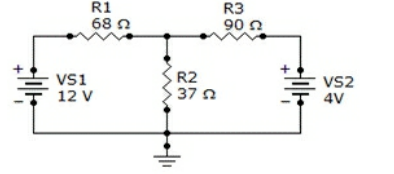(A) 850 mV
(B) 7.82 V
(C) 9.18 V
(D) 918 mV

Q 2. The branch current method is based on Kirchhoff’s voltage law and Kirchhoff’s current law.

(A) True
(B) False

Q 3. The first row of a certain determinant has the numbers 3 and 5. The second row has the numbers 7 and 2. The value of this determinant is

(A) 31
(B) -31
(C) 39
(D) -29

Q 4. Generally, the mesh current method results in fewer equations than the node voltage method.

(A) True
(B) False

Q 5. What is the voltage drop across R3?(A) 112 mV
(B) 11.25 V
(C) 180 mV
(D) 1.80 V

Q 6. Find branch current IR2.(A) 5.4 mA
(B) -5.4 mA
(C) 113.0 mA
(D) 119.6 mA

Q 7. A loop current is an actual current in a branch.

(A) True
(B) False

Q 8. The expansion method for evaluating determinants is

(A) Better than any other method
(B) Good for only one determinant
(C) More flexible than the co-factor method
(D) Good for second- and third-order determinants

Q 9. The node voltage method is based on Kirchhoff’s voltage law.

(A) True
(B) False

Q 10. In assigning the direction of branch currents,

(A) The directions are critical
(B) The directions are not critical
(C) They must point into a node
(D) They must point out of a node

Q 11. The branch current method uses

(A) Kirchhoff’s voltage and current laws
(B) Thevenin’s theorem and Ohm’s law
(C) Kirchhoff’s current law and Ohm’s law
(D) The superposition theorem and Thevenin’s theorem

Q 12. When assigning branch currents, you need not be concerned with the direction you choose.

(A) True
(B) False

Q 13. Find the node voltage VA.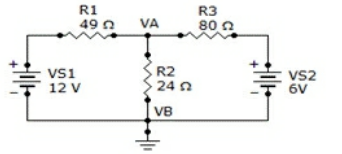(A) 6 V
(B) 12 V
(C) 4.25 V
(D) 3 V

Q 14. Second-order determinants are evaluated by subtracting the signed cross-products.

(A) True
(B) False

Q 15. Find the node voltage VA.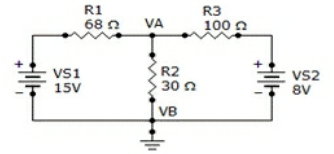(A) 518 mV
(B) 5.18 V
(C) 9.56 V
(D) 956 mV

Q 16. The mesh current method is based on Kirchhoff’s current law.

(A) True
(B) False

Q 17. Using the mesh current method, find the branch current, IR1, in the below figure.(A) 115 mA
(B) 12.5 mA
(C) 12.5 A
(D) 135 mA

Q 18. The first row of a certain determinant has the numbers 10 and 6. The second row has the numbers 3 and 5. The value of this determinant is

(A) 18
(B) 50
(C) 32
(D) -32

Q 19. The mesh method can be applied to circuits with any number of loops.

(A) True
(B) False

Q 20. Find I1.

4I1 + 4I2 = 2
6I1 + 7I2 = 4

(A) 0.5 A
(B) 50 mA
(C) -0.5 A
(D) -50 mA

Q 21. What is the current through R2?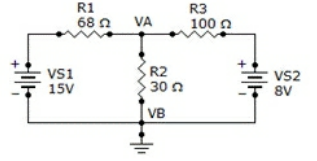(A) 3.19 A
(B) 319 mA
(C) 1.73 A
(D) 173 mA

Q 22. What is the voltage drop across R2?(A) 3.5 V
(B) 4.18 V
(C) 1.5 V
(D) 145 mV

Q 23. Find I2.

4I1 + 4I2 = 2
6I1 + 7I2 = 4

(A) 1 A
(B) -1 A
(C) 100 mA
(D) -100 mA

Q 24. Third-order determinants are evaluated by the expansion method or by the cofactor method.

(A) True
(B) False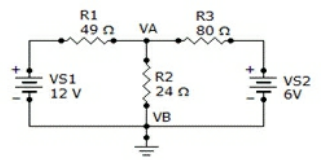(A) 177 mA